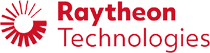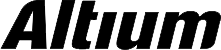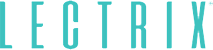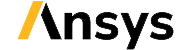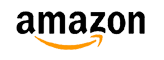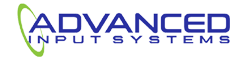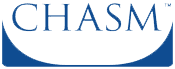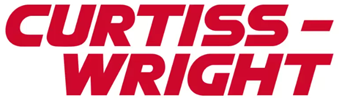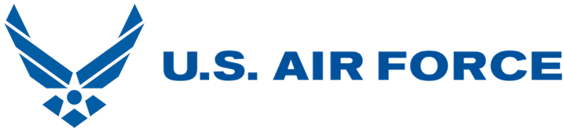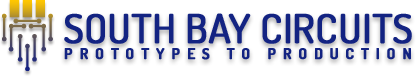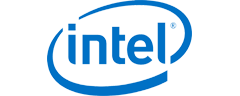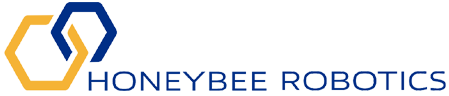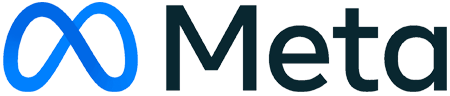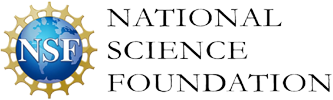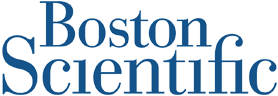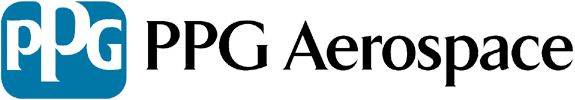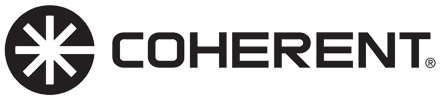﻿ Understanding Transmission Line Input Impedance and S11 | NWES Blog## Understanding Transmission Line Input Impedance and S11

By

The input impedance of a transmission line, S11, and reflection coefficients, and return loss are often confused with each other and used interchangeably. Sometimes they are the same, and sometimes they aren’t, and it depends on the specific transmission line you’re working with. The transmission line input impedance is related to S11, but it’s hard to find any resources online that state all of this so clearly.

Because of the mass confusion among many designers who need to build high speed/high frequency systems for the first time, I’ve compiled the important points to understand about transmission line input impedance. In fact, transmission line input impedance, reflection coefficients, and S11 are all related to each other. If you’re trying to size up your board and design transmission line terminations for your digital interfaces, keep reading to see how to use the input impedance.

## Transmission Line Input Impedance vs. S11

Return loss (S11) and insertion loss (S21) are two important metrics that are used to characterize broadband signal behavior in a transmission line. To get started, I’ll state very clearly that S11 is directly related to the input impedance at the input port of a circuit network, and the source impedance of the driving section of the interconnect. Consider the circuit network shown below. Here, we have four elements that must be considered when calculating S11:

• Source impedance (ZS). This is the driver with some defined source impedance (note that high speed interfaces may or may not terminate to a specific resistance).

• Load impedance (ZL). The load has its own impedance that may not be the same as the source impedance.

• Transmission line characteristic impedance (Z0). The transmission line has a characteristic impedance, but this might not be the actual impedance you’ll measure in a real experiment. Instead, we need the input impedance.

• Input impedance (Zin). The input impedance of the line depends on the characteristic impedance and the load impedance. Reflection can occur between the source impedance and the input impedance, and these values are used to calculate S11 in your transmission line.Reflection can occur between the source’s impedance and the transmission line input impedance when there is an impedance mismatch. Therefore, we need to use the input impedance to calculate reflection, which is how we define S11.

### Input Impedance Formula for a Transmission Line

The transmission line input impedance is related to the load impedance and the length of the line, and S11 also depends on the input impedance of the transmission line. The formula for S11 treats the transmission line as a circuit network with its own input impedance, which is required when considering wave propagation into an electrically long circuit network like a transmission line. The equations below define S11 in terms of the transmission line input impedance.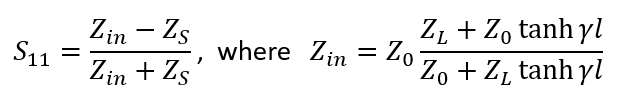Definition of S11 at the input port of a transmission line, and the transmission line’s input impedance.

Here, S11 is defined as a reflection coefficient, but it considers propagating wave behavior at the input port of the transmission line. We’ve made no assumption on the length of the line, or on the value of the source, load, and characteristic impedances. In short, this is a complete formulation of S11 for any transmission line with any source or load impedances. This same definition of S11 extends to other circuit networks that may have some other input impedance formula.

The confusion regarding S11 arises due to unstated assumptions in the definition, and I have not seen a great reference that provides a very clear definition. Unfortunately, most transmission line discussions define S11 and the reflection coefficient assuming an infinitely long line with equal source and load impedances. Not only is this extremely confusing, it’s also an idealized situation that does not reflect reality in modern digital systems (I’ll briefly discuss this more below).

### Reducing to S-parameters

Using the two equations above, we can rewrite S11 in terms of the source, load, and characteristic impedances of the transmission line. Simply substitute the input impedance equation into the S11 equation and do some simplification: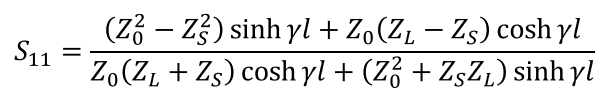General formula for S11 with any source and load impedance.

The presence of the sinh and cosh terms give rise to the resonances seen in a typical transmission line return loss spectrum. When we take ZS = ZL, the above equation takes the same form as the ABCD to S-parameter conversion equation that is found in some references. Finally, if we take the limit as the line length goes to infinity, we have Zin -> Z0, and S11 reduces to the typical reflection coefficient. This is because the wave simply has to propagate too far before it can interact with the load, and for practical purposes the load can be considered in isolation from the source. I’ll leave this as an exercise for readers.

### Designing Real Transmission Lines for High Speed Digital Interfaces

We calculated S11 above, but we can take the same approach in the opposite propagation direction to calculate S22. To get S22, simply swap ZS and ZL in the above equations and run the calculation. Thanks to reciprocity and symmetry in the interconnect structure, you now know exactly how signals will travel on the line in both directions. The S11 spectrum is quite important for designing high speed digital channels to provide sufficient bandwidth for your signals. The remaining challenge in a digital channel is to configure equalization in the receiver so that signals at the receiver can be reconstructed.

In order to get truly accurate S11 and S21 spectra for a transmission line, we need to have accurate models for characteristic impedance. The transmission characteristic impedance needs to include all sources of loss and impedance:

• Dielectric losses and dispersion. All dielectrics exhibit some dielectric loss spectrum and dispersion, which need to be included in the characteristic impedance calculation.

• Copper roughness and skin effect. The skin effect creates some resistive and inductive impedance in the characteristic impedance. Rough copper increases the skin effect impedance and has a complex enhancement factor.

• Load capacitance. Real integrated circuits have some parasitic shunt impedance at the input pin. For bi-directional channels, the input also has some load impedance. This causes real transmission lines to behave like low-pass filters up to 10’s of GHz.

If you’re engineering a high speed digital system or high frequency analog system, the layout can be difficult to create without accounting for points like transmission line input impedance. The right design firm can help you create a high-quality layout that passes basic signal integrity checks and has S11 values within application specs. I use the following formula from my recent IEEE EPEPS paper and PCB West presentation to calculate a causal characteristic impedance of transmission lines, which accounts for dispersion and copper roughness losses in terms of the characteristic impedance without losses and dispersion: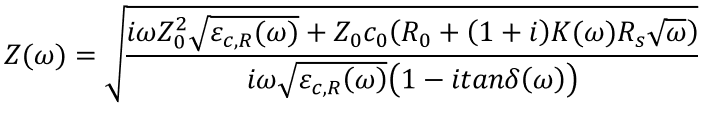Causal transmission line characteristic impedance.

Finally, we have the causal propagation constant of the channel while accounting for losses and dispersion: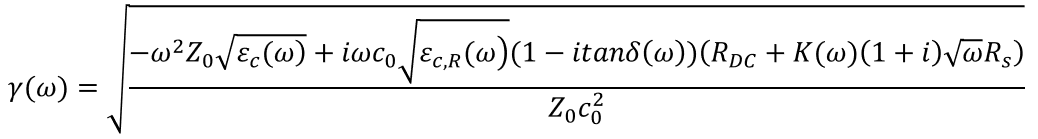Causal transmission line propagation constant.

At NWES, we provide PCB design and layout services to startups, large enterprises, and aerospace and defense electronics companies. We also design modular embedded systems that require accurate transmission line input impedance calculations and models. Our customizable product line includes advanced RF systems, high speed backplanes, power systems, and AI-capable IoT platforms. We’ve also partnered directly with EDA companies and multiple ITAR PCB manufacturing firms, and we help our clients get through the PCB manufacturing process with ease. Contact NWES for a consultation.

* indicates required

## Our Clients and Partners